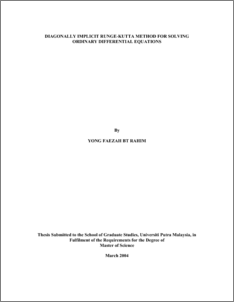# Block Diagonally Implicit Runge-Kutta Method for Solving Ordinary Differential Equations

## Citation

Rahim, Yong Faezah (2004) Block Diagonally Implicit Runge-Kutta Method for Solving Ordinary Differential Equations. Masters thesis, Universiti Putra Malaysia.

## Abstract

Block diagonally implicit Runge-Kutta (BDIRK) method of second order is derived using Butcher analysis and equi-distribution of errors (EDE) approach to outperform standard Runge-Kutta formulae. BDIRK method produces simultaneously approximation to the solution of the initial value problem (IVP) at a block of two points and . Numerical results are given to illustrate the performance of the method and comparison is made to the standard Runge-Kutta method. In this thesis, we also derived block method using embedded diagonally implicit Runge-Kutta method. The conventional one step embedded diagonally implicit Runge-Kutta (DIRK) 3(2) method which is known to be reliable and has been tested extensively is used to approximate the solution at the first point of the block, and also to generate the solution at the second point of the block. The efficiency of the method is supported by some numerical results. Finally, block embedded diagonally implicit Runge-kutta method is extended from approximating solutions at two points to three points simultaneously by increasing the order of the method. The results indicate that the block methods are competitive, both in terms of efficiency and reliability with conventional Runge-Kutta method. The stability polynomial for all the methods are obtained and their regions of stability are presented. Based on the numerical results and the stability regions it can be said that the method is suitable for solving stiff differential equations.Preview
PDF
549720_FSAS_2004_14.pdfView Item